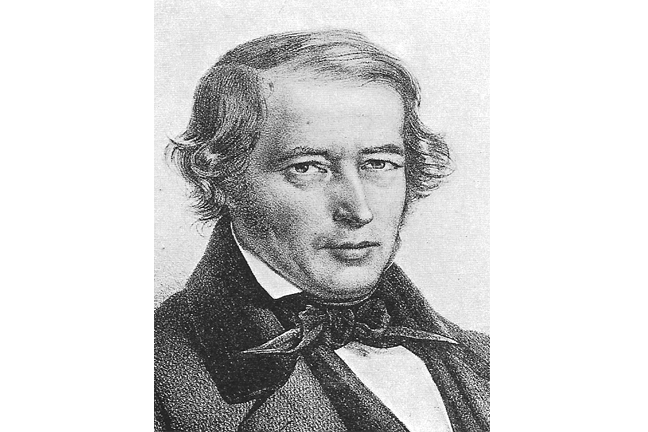4.10

## UNSW SydneyJakob Steiner

# Steiner's regions of space problem

Jakob Steiner was a prominent Swiss geometer who made many remarkable contributions to mathematics. In 1826 he asked: what is the maximum number of regions you could divide the plane into with $\normalsize{n}$ lines? And what is the maximum number of regions you could divide space into with $\normalsize{n}$ planes?

In this step we will look at both questions, and see how functions involving an exponential aspect are involved.

## How many regions of the plane with $\normalsize{n}$ lines?

Clearly with $\normalsize{0}$ lines we have $\normalsize{1}$ region, with $\normalsize{1}$ line we have $\normalsize{2}$ regions, with $\normalsize{3}$ lines we have $\normalsize{7}$ regions. After that one has to look more carefully at the alternatives: with $\normalsize{4}$ lines we have $\normalsize{11}$ regions and here we see that with $\normalsize{5}$ lines we can have $\normalsize{16}$ regions.In general, the answer is that with $\normalsize{n}$ lines you can create

regions.

Here are some small values :

 $$\normalsize{n}$$ 0 1 2 3 4 5 6 7 8 $$\normalsize{a(n)}$$ 1 2 4 7 11 16 22 29 37

Here is a graph of the function $\normalsize{a(x)=\frac{x^2+x+2}{2}}$:Q1 (M): Can you arrange $\normalsize{6}$ lines in the plane to make $\normalsize{22}$ regions?

Q2 (M): Can you see the pattern of these numbers? Could you predict the value for $\normalsize{n=9}$ without the formula?

## The regions of space problem

There is a corresponding question in space, where we may use planes to divide up space into regions. If we have $\normalsize{n}$ planes, how many regions may we create? Steiner posed and solved this problem, and showed that the answer is a cubic polynomial in $\normalsize{n}$:

Here are some small values:

 $$\normalsize{n}$$ 0 1 2 3 4 5 6 7 8 $$\normalsize{b(n)}$$ 1 2 4 8 15 26 42 64 93

Here is a graph of the function $\normalsize{b(x)=\frac{x^3+5x+6}{6}}$:Of course even within mathematics, interesting relations occur frequently. Mathematics not only answers questions, it also provides a lot of interesting questions of its own.

Q3 (C): Can you see a pattern with these numbers that would allow you to extend the table without knowing the formula? What for example is the next number in the pattern?A2. If we look at successive differences, you see that they grow by one with each step. So the difference between the 8th value and the 9th value ought to be one more than the difference between the 7th value and the 8th value, which is $\normalsize{37-29=8}$. That means the 9th value ought to be $\normalsize{37+9=46}$.
A3. The trick is to look at the differences between successive elements in the sequence. These differences are $\normalsize{1,2,4,7,11,16,22,29,\dots}$ Can you see a pattern here? The trick is to look at successive differences once again! These are $\normalsize{1,2,3,4,5,6,7,\dots}$ Now the pattern is clearer. The next difference of differences must be $\normalsize{8}$, so the next difference must be $\normalsize{37}$, so the next number must be $\normalsize{93+37=130}$. You can check the formula!